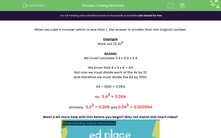# Cubing Decimals

In this worksheet, students cube simple decimal numbers.Key stage:  KS 3

Curriculum topic:   Number

Curriculum subtopic:   Understand Integer Powers/Real Roots

Difficulty level:#### Worksheet Overview

When we cube a number which is less than 1, the answer is smaller than the original number.

Example

Work out (0.4)3

We must calculate 0.4 x 0.4 x 0.4

We know that 4 x 4 x 4 = 64

But now we must divide each of the 4s by 10

and therefore we must divide the 64 by 1000.

64 ÷ 1000 = 0.064

So,  0.43 = 0.064

Similarly,  0.23 = 0.008 and 0.043 = 0.000064

Want a bit more help with this before you begin? Why not watch this short video?

### What is EdPlace?

We're your National Curriculum aligned online education content provider helping each child succeed in English, maths and science from year 1 to GCSE. With an EdPlace account you’ll be able to track and measure progress, helping each child achieve their best. We build confidence and attainment by personalising each child’s learning at a level that suits them.

Get started